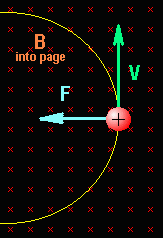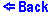Charged Particles Moving in a Magnetic Field
B is the magnetic field directed away from us. Positively charged particles are red. Negatively charged particles are blue. The green arrow shows the velocity of the particle. The gray arc is the trajectory of the particle.In this demonstration particles are entering the region of the magnetic field with their velocoties being perpendicular to the magnetic field lines. In such a situation the magnetic force serves to move the particles in a circular path. According to the "right hand rule" the magnetic force acting on the particle always remains perpendicular to its velocity.

The magnitude of the magnetic force is

F = q V B ,
where q is the magnitude of the charge of the particle, V its velocity, and B is the magnetic field.

This force can be also considered as the centripetal force

Fc = m V2 / R ,
where m is the particle's mass and R is the radius of the circular tragectory.

Making two above expressions equal to each other and solving the resulting equation for R we can easily find that the radius R of the circular path is proportional to the velocity of the particle.

R = m V / q B .© Copyright 1997, Sergey Kiselev and Tanya Yanovsky-Kiselev
Last modified: June 20, 1997